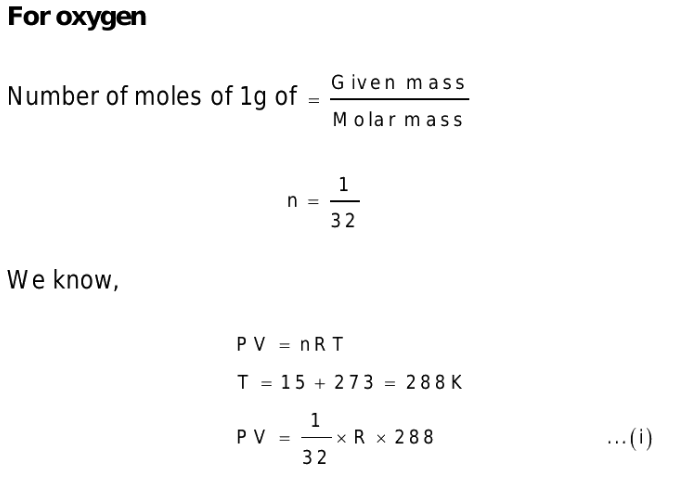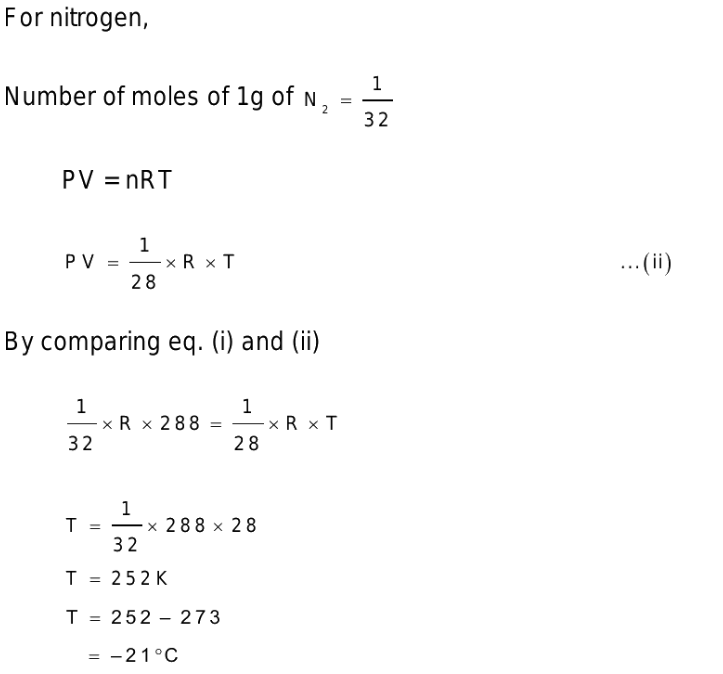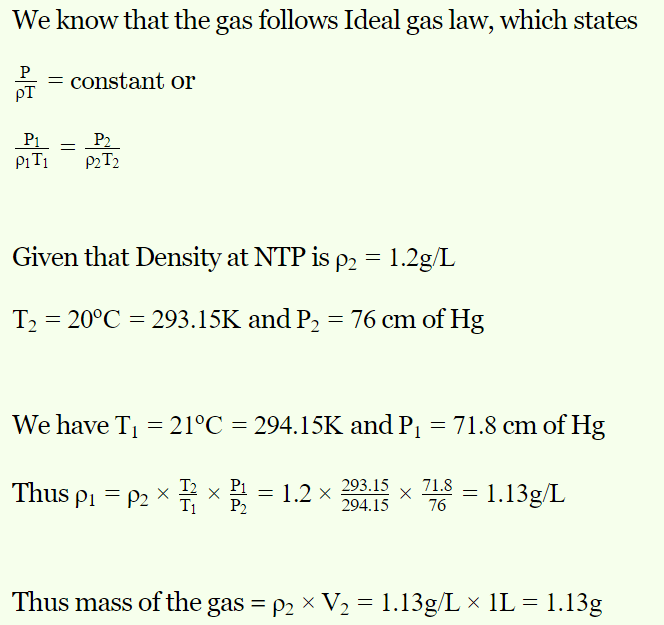## Kinetic Theory Questions and Answers Part-4

1. The equation for an ideal gas is PV = RT, where V represents the volume of
a) 1 gm gas
b) Any mass of the gas
c) One gm mol gas
d) One litre gas

Explanation: The equation for an ideal gas is PV = RT, where V represents the volume of One gm mol gas

2. The molecular weights of $O_{2}$ and $N_{2}$ are 32 and 28 respectively. At 15°C, the pressure of 1 gm $O_{2}$ will be the same as that of 1 gm $N_{2}$ in the same bottle at the temperature
a) – 21°C
b) 13°C
c) 15°C
d) 56.4°C

Explanation:3. At 100 K and 0.1 atmospheric pressure, the volume of helium gas is 10 litres. If volume and pressure are doubled, its temperature will change to
a) 400 K
b) 127 K
c) 200 K
d) 25 K

Explanation:4. Under which of the following conditions is the law PV = RT obeyed most closely by a real gas
a) High pressure and high temperature
b) Low pressure and low temperature
c) Low pressure and high temperature
d) High pressure and low temperature

Explanation: Low pressure and high temperature

5. The gas equation $\frac{PV}{T} =$ constant is true for a constant mass of an ideal gas undergoing
a) Isothermal change
c) Isobaric change
d) Any type of change

Explanation: The gas equation $\frac{PV}{T} =$ constant is true for a constant mass of an ideal gas undergoing any type of change

6. That gas cannot be liquified
a) Which obeys Vander Waal's equation
b) Which obeys gas equation at every temperature and pressure
c) The molecules of which are having potential energy
d) Which is a inert gas

Explanation: Which obeys gas equation at every temperature and pressure

7. At NTP the mass of one litre of air is 1.293 gm. The value of specific gas constant will be
a) 0.29 J/K-gm
b) 4.2 J/K-gm
c) 8.3 J/K-gm
d) 16.5 J/K-gm

Explanation: 0.29 J/K-gm

8. The volume of a gas at 21°C temperature and 768 mm pressure is 1 litre. If the density of the gas is 1.2 gm/litre at NTP, then its mass will be
a) 4 gm
b) 4.21 gm
c) 1.13 gm
d) 10 gm

Explanation:9. For one gram mol of a gas, the value of R in the equation PV = RT is nearly
a) 2 cal/K
b) 10 cal/K
c) 0.2 cal/K
d) 200 cal/K

Explanation: For one gram mol of a gas, the value of R in the equation PV = RT is nearly 2 cal/K

10. The number of molecules in a gas at pressure $1.64\times10^{-3}$   atmospheres and temperature 200 K having the volume 1 cc are
a) $6.02\times10^{16}$
b) $2.63\times10^{16}$
c) $3.01\times10^{19}$
d) $12.04\times10^{19}$